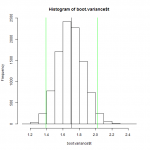Want to share your content on R-bloggers? click here if you have a blog, or here if you don't.Statistics are often taught in school by and for people who like Mathematics. As a consequence, in those class emphasis is put on leaning equations, solving calculus problems and creating mathematics models instead of building an intuition for probabilistic problems. But, if you read this, you know a bit of R programming and have access to a computer that is really good at computing stuff! So let’s learn how we can tackle useful statistic problems by writing simple R query and how to think in probabilistic terms.

In the first two part of this series, we’ve seen how to identify the distribution of a random variable by plotting the distribution of a sample and by estimating statistic. We also seen that it can be tricky to identify a distribution from a small sample of data. Today, we’ll see how to estimate the confidence interval of a statistic in this situation by using a powerful method called bootstrapping.

Answers to the exercises are available here.

Exercise 1
Load this dataset and draw the histogram, the ECDF of this sample and the ECDF of a density who’s a good fit for the data.

Exercise 2
Write a function that takes a dataset and a number of iterations as parameter. For each iteration this function must create a sample with replacement of the same size than the dataset, calculate the mean of the sample and store it in a matrix, which the function must return.

Exercise 3
Use the `t.test()` to compute the 95% confidence interval estimate for the mean of your dataset.

Learn more about bootstrapping functions in the online course Structural equation modeling (SEM) with lavaan. In this course you will learn how to:

• Learn how to develop bootstrapped confidence intervals
• Go indepth into the lavaan package for modelling equations
• And much more

Exercise 4
Use the function you just wrote to estimate the mean of your sample 10,000 times. Then draw the histogram of the results and the sampling mean of the data.

The probability distribution of the estimation of a mean is a normal distribution centered around the real value of the mean. In other words, if we take a lot of samples from a population and compute the mean of each sample, the histogram of those mean will look like one of a normal distribution center around the real value of the mean we try to estimate. We have recreated artificially this process by creating a bunch of new sample from the dataset, by resampling it with replacement and now we can do a point estimation of the mean by computing the average of the sample of means or compute the confidence interval by finding the correct percentile of this distribution. This process is basically what is called bootstrapping.

Exercise 5
Calculate the value of the 2.5 and 97.5 percentile of your sample of 10,000 estimates of the mean and the mean of this sample. Compare this last value to the value of the sample mean of your data.

Exercise 6
Bootstrapping can be used to compute the confidence interval of all the statistics of interest, but you don’t have to write a function for each of them! You can use the `boot()` function from the library of the same name and pass the statistic as argument to compute the bootstrapped sample. Use this function with 10,000 replicates to compute the median of the dataset.

Exercise 7
Look at the structure of your result and plot his histogram. On the same plot, draw the value of the sample median of your dataset and plot the 95% confidence interval of this statistic by adding two vertical green lines at the lower and higher bounds of the interval.

Exercise 8
Write functions to compute by bootstrapping the following statistics:

• Variance
• kurtosis
• Max
• Min

Exercise 9
Use the functions from last exercise and the boot function with 10,000 replicates to compute the following statistics:

• Variance
• kurtosis
• Max
• Min

Then draw the histogram of the bootstrapped sample and plot the 95% confidence interval of the statistics.

Exercise 10
Generate 1000 points from a normal distribution of mean and standard deviation equal to the one of the dataset. Use the bootstrap method to estimate the 95% confidence interval of the mean, the variance, the kurtosis, the min and the max of this density. Then plot the histograms of the bootstrap samples for each of the variable and draw the 95% confidence interval as two red vertical line.

Two bootstrap estimate of the same statistic of two sample who are distributed by the same density should be pretty similar. When we compare those last plots with the confidence interval we drawn before we see that they are. More importantly, the confidence interval computed in exercise 10 overlap the confidence interval of the statistics of the first dataset. As a consequence, we can’t conclude that the two sample come from different density distribution and in practice we could use a normal distribution with a mean of 0.4725156 and a standard deviation of 1.306665 to simulate this random variable.